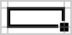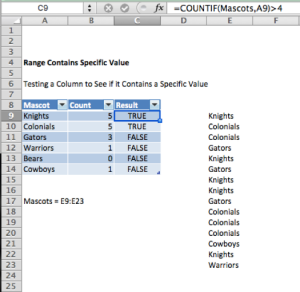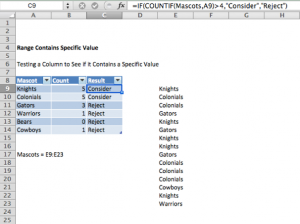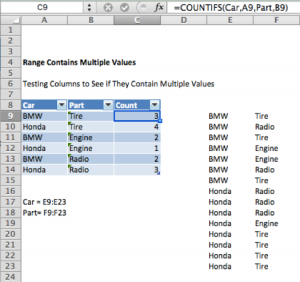### Test if Cell Contains Specific Value

In this tutorial you will learn how to test if a range contains a specific value.

## COUNTIF Function

In Excel, the COUNTIF function is used to determine if a value exists in a range of cells. The general formula for the COUNTIF is as follows:

=COUNTIF(range, criteria)

Range is the group of cells that you want to count. They can contain numbers, arrays, be named, or have references that contain numbers.

Criteria is a number (5), expression (>5), cell reference (A9), or word (Knights) that determines which cells to count.

We will use the COUNTIF function to solve the following example.

## Example: Countif Cells Contain Certain Text

Suppose a school wants to change their team mascot. They give 6 different options to student council officials and decide that only mascots with more than 4 votes will be considered. What mascots will the school consider?

Part 1) First we have to have to determine how many votes each mascot got. To do so we use the following formula:

Range E9:E23 was renamed to “Mascots” for simplicity purposes using a Named Range

This will count the number of cells in the list of Mascots that match the value of A9. The result is 5. To quickly fill in the remaining cells you can simply select the cell that you want to use as a basis and drag the fill handle.

## Alternative Expressions for the formula used

• =COUNTIF(E9:E23, “Knights”) Will count the number of cells with Knights in cells E9 through E23. The result is 5.
• =COUNTIF(E9:E23, “?nights”) Will count the number of cells that have exactly 7 characters and end with the letters “nights” in cells E9:E23. The question mark (?) acts as a wildcard to replace an individual character. The result is 5.
• =COUNTIF(E9:E23,”<>”&”Knights”) Will count the number of cells in the range E9 through E23 that don’t contain Knights. The result is 10.

Part 2) Next, we have to determine which mascots received more than 4 votes. To do so we will use the COUNTIF function to run a simple TRUE or FALSE test.

=COUNTIF(Mascots, A9)>4This formula will produce the answer TRUE if the desired mascot appears in the range more than 4 times and FALSE if it appears less than or equal to 4 times. Once again we can select the cell that we want to use as a basis and drag the fill handlein order to fill the remaining cells.

## Manipulating the COUNTIF Function

We can also wrap the formula inside an IF statement in order to produce a result different than TRUE or FALSE.

=IF(COUNTIF(Mascots,A9)>4,”Consider”,”Reject”)Instead of producing the result TRUE or FALSE, this formula will cause Excel to produce the result Consider or Reject.

## COUNTIFS Function with Multiple Criteria

Once you learn how to use the COUNTIF function with one range and criteria, it is easy to use it for two or more range/criteria combinations. When testing if a range contains multiple values we must use the COUNTIFS function.

## EXAMPLE

Let’s say we have a list of car parts ordered. We want to count the number of times a car part was ordered for its corresponding vehicle.By using the general formula:

=COUNTIFS(range1,criteria1,range2,criteria2)

We are able to determine where both criteria are matched in their respective columns. Let it be noted that the two criteria ranges must be the same shape and size or the formula will not work.